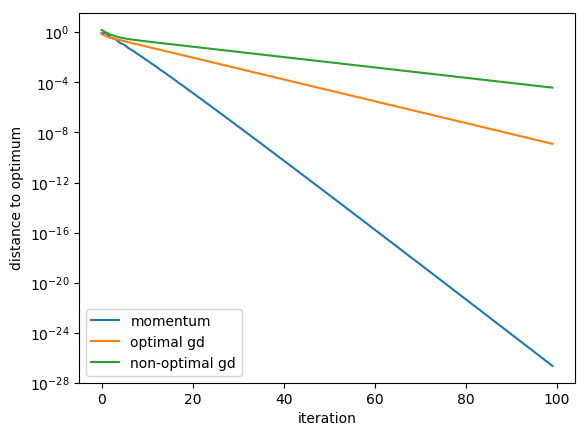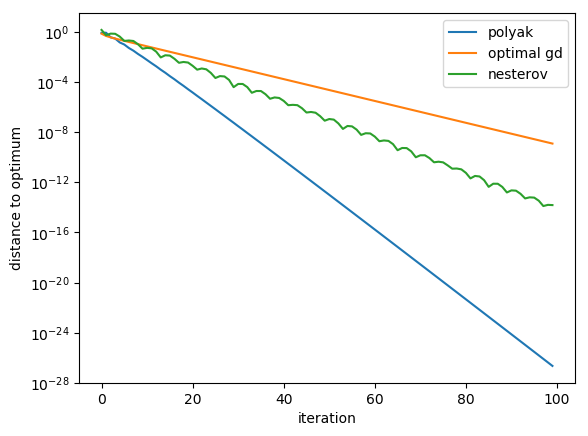In :
using PyPlot
using LinearAlgebra
using Random

Random.seed!(8765309);


## Analysis of Momentum¶

In :
function gradient_descent(w0, A, alpha, niters)
w = w0
rv = zeros(niters)
for i = 1:niters
w = w - alpha * A * w
rv[i] = norm(w)
end
return rv
end

function momentum_gd(w0, A, alpha, beta, niters)
w = w0
wprev = w0
rv = zeros(niters)
for i = 1:niters
(w, wprev) = (w - alpha * A * w + beta * (w - wprev), w)
rv[i] = norm(w)
end
return rv
end

Out:
momentum_gd (generic function with 1 method)
In :
# quadratic objective with many different eigenvalues and kappa = 10
A = diagm(0=>[0.1,0.2,0.3,0.4,0.5,0.6,0.7,0.8,0.9,1.0]);
beta = ((sqrt(10) - 1)/(sqrt(10) + 1))^2;
alpha_mom = (2 + 2*beta) / (1 + 0.1);
alpha_gd = 2 / (1 + 0.1);

In :
w0 = randn(10);

In :
dists_gd = gradient_descent(w0, A, alpha_gd, 100);
dists_gd2 = gradient_descent(w0, A, 0.5*alpha_gd, 100);
dists_mom = momentum_gd(w0, A, alpha_mom, beta, 100);

In :
semilogy(dists_mom; label="momentum");
semilogy(dists_gd; label="optimal gd");
semilogy(dists_gd2; label="non-optimal gd");
legend();
xlabel("iteration");
ylabel("distance to optimum");In :
function nesterov_gd(w0, A, alpha, beta, niters)
w = w0
v = w0
rv = zeros(niters)
for i = 1:niters
wprev = w
w = v - alpha * A * v
v = w + beta * (w - wprev)
rv[i] = norm(w)
end
return rv
end

Out:
nesterov_gd (generic function with 1 method)
In :
beta_nest = (sqrt(10) - 1)/(sqrt(10) + 1);
alpha_nest = 1;
dists_nest = momentum_gd(w0, A, alpha_nest, beta_nest, 100);

In :
semilogy(dists_mom; label="polyak");
semilogy(dists_gd; label="optimal gd");
semilogy(dists_nest; label="nesterov");
legend();
xlabel("iteration");
ylabel("distance to optimum");